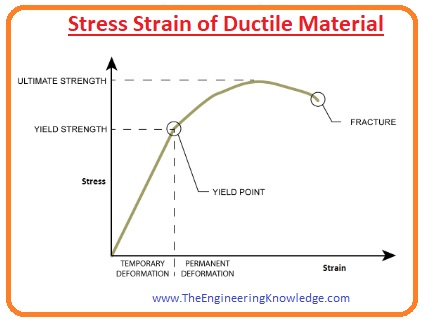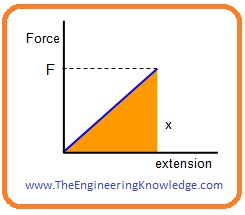Hello dear FSC students, I hope you all are doing great. In today’s tutorial, we will have a look at 2nd Year Physics Chapter 17 Solved Questions. I have started a series of tutorials related to the solution of questions given in 2nd-year physics. In the previous tutorial, I have discussed all the questions of chapter16 with the detailed.

In this post, we will have a detailed look at all the questions given in chapter 17 of FSC physics. I will explain all the questions one by one and give an answer to every question in a simple way. So let’s get started with 2nd Year Physics Chapter 17 Exercise Solved Questions.

#### 2nd Year Physics Chapter 17 Exercise Solved Questions

• So friends let’s discuss all these questions one by one with detailed.

#### Question 17.1

Crystalline Solids:

• Such solids in which atoms, molecules are arranged at a regular pattern called crystalline solids.
• The compounds like sodium chloride and ceramics material are crystalline solids,
• When any force is applied on crystalline solids their atoms and molecules are vibrated continuously about their center point with specific amplitude.
• Their vibration amplitude increases with the increment in temperature.
• Irrespective vibrations their atoms, ions, and molecules retain their positions with the cohesive force.
• For each crystalline solid there is specif temperature over which the vibrations of its atoms increase and they melt.

Amorphous Solids:

• the solids that have no special pattern for atoms, ions, and molecules are called amorphous solids. These solids also are known as glassy solid.
• Due to the irregular pattern of atoms, these solids are like the liquid with the disordered pattern in frozen shape.
• An example of these solids is glass that is irregular arrangements of atoms at normal temperature.
• For these solids, there is no specific melting point due to irregular arrangements.

Polymeric solids:

• There are such solids that have properties of solids and non-solids is called polymeric solids.
• These solids are also known as partially crystalline solids. The plastic and synthetic rubber are known as a polymer.

#### Question 17.2:

Stress:

• The force applied on the unit area to change the shape, volume and length of that body are called stress.
• The mathematical expression for stress is given below.

Stress = Force/ Area = F/A

• From the above equation, you can see that the unit of stress is newton meter or Pascal.

Strain:

• After the application of stress the variation in length, volume or shape of the body is called strain.

Tensile Stress:

• If after application of stress length of the body varies it known as tensile stress.

Tensile Strain:

• The fractional change in length after application of stress is called tensile strain.

Compressive stress:

• The force on per unit area is called stress.

Compressive Strain:

• The strain generated due to the compressive stress is known as compressive strain.

Shear stress:

• The angular deformation due to the application of force is called shear stress.

Shear strain:

• Due to angular deformation shear strain occurs. It is the result of shear stress.

#### Question 17.3:

Modulus of Elasticity:

• The ration between stress applied to the body to strain is called modulus of elasticity. Thre are three types of modulus of elasticity.

young’s Modulus:

• The ration between tensile stress to tensile strain is known as young’s modulus.

Y=Tensile Stress / Tensile Strain

Y=(F/A)/(Δl/l)

Bulk Modulus:

• The ratio between volume stress to volume strain is called bulk strain. It mathematically expression is given here.

Bulk Modulus = Compressive Stress / Volume Strain

K=(F/A)/(ΔV/V)

Shear Modulus:

• The ratio between shear stress to shear strain is called shear modulus.

Shear Modulus = Shear Stress / Shear Strain

G=(F/A)/tanθ

Units of Modulus of Elasticity:

Stress/Strain=Nm-2 /no unit

=Nm-2 or Pascal

Unit of Stress

Force/Area= N/m2

• You can see that units of stress and modulus of elasticity are the same.

#### Question 17.4:Elastic Limit:

• The maximum stress bear by any material without any permanent variation in shape or in other dimensions is called Elastic Limit.

Yield Point:

• The point at which any substance starts to flow for example strain increases for time up to the breaking point without a further increment in stress.

Ultimate Tensile Stress:

• The extreme stress which any substance can bear it also known as the nominal strength of the material.

#### Question 17.5:

• If we follow Hook’s law then we will observe that the force-extension graph within elastic limit is a linear or straight line.
• If we stretch any solid material energy will be stored in that material.
• When deformation force varies from ‘0’ to ‘F’ then an extension is generated.
• The formula for the average force is given here.

(0 + F)/2 = F/2

• The for applied or work done stretches the solid through ‘x’ is given here.

W  = (F /2)*x

• The work done for an extension is stored in the shape of strain.#### Question 17.6:

• Electrons in an atom are bounded with the nucleus and reside in specific energy levels or shells.
• For the formation of solids when many numbers of atoms suppose that ‘n’ are come close to each other than every energy level of an atom will divide into further sub-levels that is known as states.
• The separation is done by the force applied by the other atoms.
• All these states or sub-levels are separated but they seem closely attach to make continuous energy bands.
• Among the 2 energy bands, there is some distance where is no electrons exits.
• This space is known as energy states and its range is known as a forbidden energy gap.

Conductors:

• Conductors are such material that has large number of electrons for electric conduction.
• In these substances valence and conduction bands are overlapped with each other.
•  There is no physical difference among these 2 bands that make sure the existence of large number of electrons.

Insulators:

• In these substances, electrons are very tightly bound with their atoms and there is nor free electrons for conduction.
• In insulators, the valence band is filled with electrons and an empty conduction band.
• The difference between the valence band and conduction band is large so electrons can not move from valence band to conduction band.

Semi-Conductors:

• These substances have partially filled conduction band and valence band also partially filled and forbidden gap between valence and conduction band.

#### Question 17.7:

Intrinsic semiconductor:

• The pure substance of any material is known as an intrinsic semiconductor.
• These substances have the same number of positive charge carriers hole and negative charge carrier electrons.

Extrinsic semiconductor:

• These substances have some amount of impurity material. The conductive behavior of these atoms increases according to the types and quantity of added impurity.
• If we add any element from group five of the periodic table in silicon then we will have N types substance.
• If impurity added belongs to group three elements then the resultant material is called P-type.

#### Question 17.8:

• The pure or intrinsic semiconductor substance has the same quantity of negative charge carrier electrons and positive charge carrier holes.
• After applications of voltage about the semiconductor substance electrical field is generated.
• This field provides drift velocity to the electrons and they move in the opposite direction.
• Due to the movement of electrons current flows through pure semiconductor substances.

#### Question 17.9:

• Those substances whose resistivity becomes ‘0’ at a specific value of temperature is called superconductor.
• When the resistance of substance becomes ‘0’ no loss of power and current start to flow without the absence of any voltage source.
• The temperature for which resistivity is ‘0’ known as critical temperature for example for mercury T = 4.2 Kelvin, for Aluminum Temperature is 1.18 Kelvin.
• Kmaerlingh Ornes in 1911 first time created the superconductor.

#### Question 17.10:

Paramagnetic substances:

• In paramagnetic substance, the spin and orbital axis of electrons are such arrange that their field supports one another and atoms operate like a small magnet.
• The example of these substances is manganese, aluminum, platinum, etc.

Diamagnetic substances:

• In diamagnetic substance, the field generated by the spin and orbital motion of electrons cancel each other effet.
• Example of these substances is water, copper, Antimony.

Ferromagnetic substances:

• In these substances, atoms will cooperate with one another in such a way that they exhibit strong magnetic effects. Examples of these substances is iron, Nickel, chromium dioxide.

#### Question 17.11:

• The energy required to magnetize and demagnetize any substance is called a hysteresis loop.
• The power dissipated in the form of heat is known as hysteresis loss.
• Such material that has less hysteresis loss is used for the construction of the transformer because it easily magnetizes and demagnetized.

#### Related Posts

So, friends, it is a detailed post about 2nd Year Physics Chapter 17 Exercise Solved Questions if you have any questions ask in comments. See you in next post have a good day. I will soon upload a solution of chapter 18 exercise.